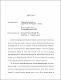## Quantum Computing for Optimization and Machine Learning2022
##### Authors
Chakrabarti, Shouvanik
Quantum Computing leverages the quantum properties of subatomic matter to enable computations faster than those possible on a regular computer. Quantum Computers have become increasingly practical in recent years, with some small-scale machines becoming available for public use. The rising importance of machine learning has highlighted a large class of computing and optimization problems that process massive amounts of data and incur correspondingly large computational costs. This raises the natural question of how quantum computers may be leveraged to solve these problems more efficiently. This dissertation presents some encouraging results on the design of quantum algorithms for machine learning and optimization. We first focus on tasks with provably more efficient quantum algorithms. We show a quantum speedup for convex optimization by extending quantum gradient estimation algorithms to efficiently compute subgradients of non-differentiable functions. We also develop a quantum framework for simulated annealing algorithms which is used to show a quantum speedup in estimating the volumes of convex bodies. Finally, we demonstrate a quantum algorithm for solving matrix games, which can be applied to a variety of learning problems such as linear classification, minimum enclosing ball, and $\ell-2$ margin SVMs. We then shift our focus to variational quantum algorithms, which describe a family of heuristic algorithms that use parameterized quantum circuits as function models that can be fit for various learning and optimization tasks. We seek to analyze the properties of these algorithms including their efficient formulation and training, expressivity, and the convergence of the associated optimization problems. We formulate a model of quantum Wasserstein GANs in order to facilitate the robust and scalable generative learning of quantum states. We also investigate the expressivity of so called \emph{Quantum Neural Networks} compared to classical ReLU networks and investigate both theoretical and empirical separations. Finally, we leverage the theory of overparameterization in variational systems to give sufficient conditions on the convergence of \emph{Variational Quantum Eigensolvers}. We use these conditions to design principles to study and evaluate the design of these systems.